# Analysis of electromagnetic characteristic in the interior permanent magnet brushless DC motor

## Zhengyang Li1, Yi Wan2, Yan Xia3, Fangbin Gong4, Qiang Wang5, Fei Chen6

1, 2Key Laboratory of High Efficiency and Clean Mechanical Manufacture, Ministry of Education, School of Mechanical Engineering, Shandong University, Jinan, China

1, 2National Demonstration Center for Experimental Mechanical Engineering Education, Shandong University, Jinan, China

3School of Mechanical Engineering, Qilu University of Technology (Shandong Academy of Sciences), Jinan, China

4, 5, 6DeZhou HengLi Electrical Machinery Co., Ltd, Dezhou, China

2Corresponding author

Mathematical Models in Engineering, Vol. 8, Issue 3, 2022, p. 91-97. https://doi.org/10.21595/mme.2022.22950
Received 22 August 2022; received in revised form 19 September 2022; accepted 27 September 2022; published 30 September 2022

Copyright © 2022 Zhengyang Li, et al. This is an open access article distributed under the Creative Commons Attribution License, which permits unrestricted use, distribution, and reproduction in any medium, provided the original work is properly cited.
Views 50
CrossRef Citations 0
Abstract.

The motor vibration is mainly induced by the electromagnetic excitation. In order to improve the running performance of the permanent magnet brushless DC motor (BLDCM) within the rolling rotor compressor, this paper builds the predicted model of the electromagnetic excitation and analyzes the electromagnetic characteristic of the BLDCM. Firstly, the electromagnetic field is divided into four regions in 2D plane, including the magnet, air gap, stator slot, and slot-opening subdomains. Then, the relative equations of the electromagnetic field are constructed, and the vector magnetic potential is solved by the variable separation method. Thus, the magnetic flux density of the air gap is derived by the corresponding vector magnetic potential, which is used to describe the electromagnetic excitation. Based on the built model, the effects of the slot opening and air gap length on the electromagnetic characteristic are analyzed. The analysis results can provide a reference for the improvement of the electromagnetic characteristic of the BLDCM by structure optimization.

Highlights
• A 3D model of BLDCM is built to analyze its sturcture.
• The electromagnetic characteristic of the BLDCM was analyzed.
• The effect of the slot opening on the magnetic flux density was analyzed.
• The effect of the air gap length on the electromagnetic force was analyzed.

Keywords: BLDCM, electromagnetic characteristic, slot opening, air gap length.

#### 1. Introduction

Due to the traits of high efficiency, easy control, long-life, simple construction and so on, the BLDCM is widely used in the various fields, especially in the home appliance . For example, the BLDCM within the rolling rotor compressor is selected as the driving motor in the air conditioning. The vibration of the BLDCM could directly affect the running performance of the overall system, which is mainly caused by the electromagnetic excitation .

The research methods for the motor electromagnetic fields mainly include the analytical method, the finite element method (FEM), the graphical method, and so on. The FEM can ensure the predicted accuracy, but it will loss the efficiency. The analytical method shows the good calculated efficiency. Such as, Zhu et al.  investigated the effect of stator slotting on the radial magnetic field distribution. Dubas et al.  developed a new analytical solution for the multipole surface mounted permanent-magnet motors, where both the radial and parallel magnetization and the slotting effect were considered. Then, Zhu et al.  built an accurate analytical subdomain model for the magnetic field computation in surface-mounted permanent-magnet machines. Additionally, Lubin et al.  also proposed an analytical subdomain model to compute the magnetic field distribution in surface-mounted permanent-magnet motors with semi-closed slots, where the expression and solution of this model show more simplified and simple. He et al.  investigated the influence of rotor eccentricity on the electromagnetic performance by the finite element analyses and experiments.

In this paper, in order to investigate the electromagnetic characteristic of the BLDCM, the method  is introduced to describe the electromagnetic field. In Section 2, the predicted model is constructed by combining the equations of the different subdomains in 2D polar coordinates, and thus the electromagnetic excitation is derived and obtained. Then, the effects of the structure parameter including the slot opening and air gap length on the electromagnetic characteristic are analyzed in Section 3. Finally, some conclusions are listed in Section 4.

#### 2.1. Physical model of BLDCM

The BLDCM in this paper is inside the rolling rotor compressor used in the refrigeration equipment, such as air conditioning, refrigerators, and so on. The motor mainly consists of the rotor, stator, rolling rotor, shaft, sliding bearing and shell, whose 3D structure is shown in Fig. 1. The rotor, rolling rotor and sliding bearing are mounted on the same rotating shaft. During running, the BLDCM drives the rotating shaft and rolling rotor to rotate, which changes the volume of the working cavity composed of the rolling rotor and air cylinder to compress gas. As the basis of analysis the dynamic characteristic of the BLDCM, the electromagnetic excitation is investigated in the next subsection.

Fig. 1. The 3D schematic diagram of the BLDCM: 1 – rolling rotor; 2 –shaft; 3 – air cylinder; 4 – upper bearing; 5 – stator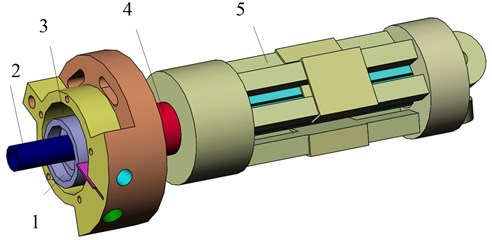#### 2.2. Analytical model of magnetic field

In Fig. 2, the cross section of the BLDCM is artificially divided into four parts, including the magnet, air gap, stator slot, and slot-opening subdomains. In 2D polar coordinates, i.e. $\left(r,\theta \right)$, ${R}_{1}$ and ${R}_{2}$ are the inner and outer radiuses of the permanent magnet, respectively. The rotor and permanent magnet subdomain are in contact at ${R}_{1}$; the two subdomains of the permanent magnet and air gap are in contact at ${R}_{2}$. And ${R}_{3}$ are the outer radius of the air gap subdomain, where the two subdomains of the air gap and slot-opening are in contact at ${R}_{3}$. ${R}_{4}$ and ${R}_{5}$ are the outer radiuses of the slot-opening and stator slot subdomains, respectively. In addition, $\alpha$ and $\beta$ are the slot-opening angle and the slot-pitch angle, respectively.

For the sake of convenient analysis, some assumptions are listed as follows: (1) the silicon steel sheets are infinitely permeable; (2) the end effect is ignored; (3) the relative permeability of permanent magnet subdomain ${\mu }_{0}\ne 1$.

In air-gap subdomain, the Laplace equation can be built in forms of polar coordinates, which can be described as:

(1)

where ${A}_{2}$ is the vector magnetic potential of air gap subdomain.

The boundary conditions of the air gap subdomain are:

(2)
$\begin{array}{l}{\frac{\partial {A}_{2}}{\partial r}|}_{r={R}_{2}}={\frac{1}{{\mu }_{0}}\frac{\partial {A}_{1}}{\partial r}|}_{r={R}_{2}},\\ {\frac{\partial {A}_{2}}{\partial r}|}_{r={R}_{3}}=\left\{\begin{array}{ll}{\frac{\partial {A}_{3}}{\partial r}|}_{r={R}_{3}},& \theta -\frac{\alpha }{2}\le \theta \le \theta +\frac{\alpha }{2},\\ 0,& \mathrm{o}\mathrm{t}\mathrm{h}\mathrm{e}\mathrm{r}\mathrm{s},\end{array}\right\\end{array}$

where ${A}_{1}$ and ${A}_{3}$ are the vector magnetic potentials of the permanent magnet and slot-opening subdomains, respectively.

Fig. 2. Schematic diagram of cross section of the BLDCM: 1 – stator; 2 – air gap; 3 – permanent magnet; 4 – slot; 5 – slot opening; 6 – rotor; 7 – shaft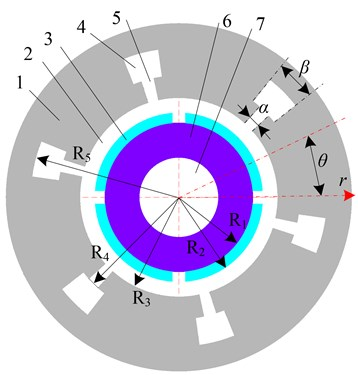Based on the variable separation method, Eq. (1) can be solved, whose general solution can be expressed by:

(3)

where ${E}_{n}$, ${F}_{n}$, ${G}_{n}$ and ${H}_{n}$ refer to the harmonic coefficients of the magnetic vector potential in the air gap. Functions $S$ and $T$ are used to deal with the solutions of the magnetic vector potential .

The relationship between the magnetic flux density and the vector magnetic potential can be described as:

(4)
${B}_{r}=\frac{1}{r}\frac{\partial A}{\partial \theta }{B}_{\theta }=-\frac{\partial A}{\partial \theta }.$

Based on the Eq. (4), the radial and tangential magnetic flux density in the air gap can be obtained by:

(5)

where:

(6)
$\begin{array}{l}{P}_{1n}=-\frac{1}{r}\left[{E}_{n}{R}_{2}\frac{{S}_{n}\left(r,{R}_{3}\right)}{{T}_{n}\left({R}_{2},{R}_{3}\right)}+{F}_{n}{R}_{3}\frac{{S}_{n}\left(r,{R}_{2}\right)}{{T}_{n}\left({R}_{3},{R}_{2}\right)}\right],\\ {P}_{2n}=-\frac{1}{r}\left[{\mathrm{G}}_{n}{R}_{2}\frac{{T}_{n}\left(r,{R}_{3}\right)}{{T}_{n}\left({R}_{2},{R}_{3}\right)}+{H}_{n}{R}_{3}\frac{{T}_{n}\left(r,{R}_{2}\right)}{{T}_{n}\left({R}_{3},{R}_{2}\right)}\right],\\ {P}_{3n}=\frac{1}{r}\left[{G}_{n}{R}_{2}\frac{{S}_{n}\left(r,{R}_{3}\right)}{{T}_{n}\left({R}_{2},{R}_{3}\right)}+{H}_{n}{R}_{3}\frac{{S}_{n}\left(r,{R}_{2}\right)}{{T}_{n}\left({R}_{3},{R}_{2}\right)}\right],\\ {P}_{4n}=-\frac{1}{r}\left[{E}_{n}{R}_{2}\frac{{T}_{n}\left(r,{R}_{3}\right)}{{T}_{n}\left({R}_{2},{R}_{3}\right)}+{F}_{n}{R}_{3}\frac{{T}_{n}\left(r,{R}_{2}\right)}{{T}_{n}\left({R}_{3},{R}_{2}\right)}\right],\end{array}$

where $n$ is a positive integer.

The electromagnetic torque can be expressed by:

(7)

where ${L}_{m}$ is the axial length of the stator core, and ${\mu }_{0}$ is the air gap permeability.

Substitution Eq. (5) into Eq. (7), the electromagnetic torque can be described by the Fourier series:

(8)
${T}_{e}=\frac{\pi {L}_{m}{r}^{2}}{{\mu }_{0}}\sum _{n=1}^{\infty }\left({P}_{1n}{P}_{2n}{P}_{3n}{P}_{4n}\right).$

Based on the Maxwell tensor formula, the electromagnetic force along $x$ and $y$ directions can be written as:

(9)
$\begin{array}{l}{F}_{ex}=\frac{{L}_{m}r}{2{\mu }_{0}}{\int }_{0}^{2\pi }\left[\left({B}_{2r}^{2}-{B}_{2\theta }^{2}\right)\mathrm{c}\mathrm{o}\mathrm{s}\theta -2{B}_{2r}{B}_{2\theta }\mathrm{s}\mathrm{i}\mathrm{n}\theta \right]d\theta ,\\ {F}_{ey}=\frac{{L}_{m}r}{2{\mu }_{0}}{\int }_{0}^{2\pi }\left[\left({B}_{2r}^{2}-{B}_{2\theta }^{2}\right)\mathrm{s}\mathrm{i}\mathrm{n}\theta +2{B}_{2r}{B}_{2\theta }\mathrm{c}\mathrm{o}\mathrm{s}\theta \right]d\theta .\end{array}$

Then, substituting the magnetic flux density in Eq. (5) into Eq. (9), Eq. (9) can be rewritten as the follows:

(10)

#### 3. Analysis of electromagnetic characteristic of the BLDCM

This paper takes the BLDCM in the rolling rotor compressor of an air conditioner as an example, where the corresponding system parameters are listed in Table 1. Then, the system parameters are substituted into the above equations to obtain the electromagnetic excitation. The effects of the air gap length and the slot opening on the electromagnetic characteristic are analyzed in detail.

Table 1. Parameters of the BLDCM

 Items Value Items Value Axial length of stator ${L}_{m}$ 80 (mm) Pole-arc coefficient 1 Outer radius of rotor ${R}_{1}$ 24.6 (mm) Pole-pairs number 2 Outer radius of magnet ${R}_{2}$ 27.7 (mm) Number of stator slots 6 Radius of stator bore ${R}_{3}$ 28.9(mm) Relative permeability of magnet ${\mu }_{0}$ 1.02 Radius of slot opening ${R}_{4}$ 31 (mm) Residual magnetic flux density ${B}_{r}$ 1.2 T Radius of slot ${R}_{5}$ 46.4 (mm) Speed of motor 3000 r/min

#### 3.1. The effect of the slot opening on the magnetic flux density (MFD)

In order to analyze the effect of the slot opening ($\alpha$/$\beta$) on the magnetic flux density (MFD), three values of the slot opening are selected, including $\alpha$/$\beta$ = 0.1, 0.3 and 0.5. The radial and tangential MFDs of the air gap are illustrated in the Fig. 3. In the figure, when the slot opening increases from 0.1 to 0.5, the fluctuation of magnetic flux density gradually aggravates. Especially, the radial MFDs obviously fluctuate at the slot opening. This shows that the fluctuation resulted from slot opening directly affects the electromagnetic excitation.

Fig. 3. Magnetic flux density of air gap under different slot opening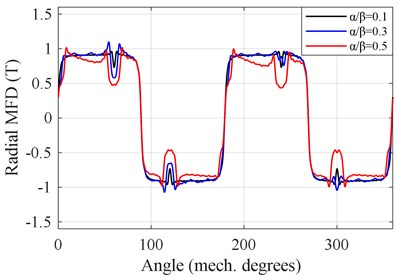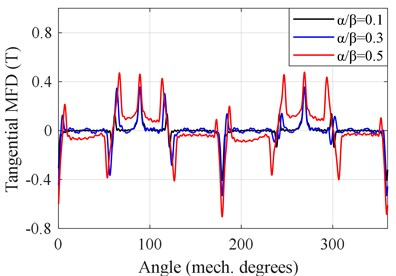b) Tangential MFD

#### 3.2. The effect of the air gap length on the electromagnetic force

Under the different air gap length, the rotor is subjected to the different electromagnetic forces, which further affects the dynamic characteristic of the motor. The air gap length (AGL) is selected with 1 mm, 2 mm and 3 mm, where the slot opening is 0.1. Then, the obtained electromagnetic forces (EFs) along $x$ and $y$ directions are shown in Fig. 4. When the air gap length increases, the electromagnetic forces gradually decreases. In other words, the increasing air gap length would weaken the electromagnetic excitation. Therefore, the structural parameters of the motors, such as the slot opening and the air gap length, should be optimized to improve the electromagnetic characteristic.

Fig. 4. Electromagnetic force under different air gap length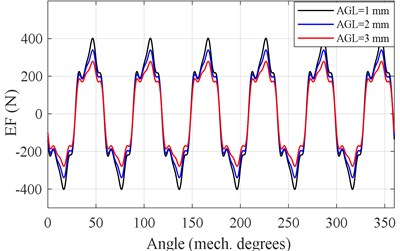a) EF along $x$ direction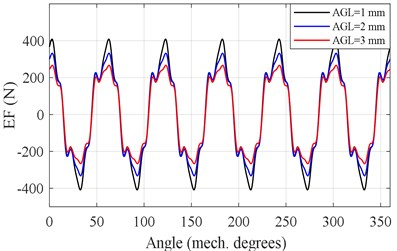b) EF along $y$ direction

#### 4. Conclusions

In this paper, a predicted model of the electromagnetic excitation for the BLDCM is built. Based on the obtained the vector magnetic potential, magnetic flux density of the air gap is derived, and thus the electromagnetic excitation including the electromagnetic torque and force are described. Then, the electromagnetic characteristics are analyzed according to the built model. The analysis results show that the increasing slot opening will aggravate the fluctuation of magnetic flux density, but the increasing air gap length can weaken the electromagnetic force. Therefore, the electromagnetic characteristic of the motor can be optimized using the appropriate structural parameters.

#### Acknowledgements

This work was supported by the National Natural Science Foundation of China (Grant No. 51975336), Key Research and Development Program of Shandong Province (Grant No. 2020JMRH0202), the New Old Energy conversion Major Industrial Tackling Project of Shandong Province (Grant No. 2021-13), the Education and Teaching Reform Research Project of Shandong University (2022Y133, 2022Y124, 2022Y312), and Key Research and Development Program of Jining City (2021DZP005).

1. M. F. Unlersen, S. Balci, M. F. Aslan, and K. Sabanci, “The speed estimation via BiLSTM-based network of a BLDC motor drive for fan applications,” Arabian Journal for Science and Engineering, Vol. 47, No. 3, pp. 2639–2648, Mar. 2022, https://doi.org/10.1007/s13369-021-05700-w [Publisher]
2. A. Zhang, Y. Bai, B. Yang, and H. Li, “Analysis of nonlinear vibration in permanent magnet synchronous motors under unbalanced magnetic pull,” Applied Sciences, Vol. 8, No. 1, p. 113, Jan. 2018, https://doi.org/10.3390/app8010113 [Publisher]
3. Z. Q. Zhu and D. Howe, “Instantaneous magnetic field distribution in brushless permanent magnet DC motors. III. Effect of stator slotting,” IEEE Transactions on Magnetics, Vol. 29, No. 1, pp. 143–151, Jan. 1993, https://doi.org/10.1109/20.195559 [Publisher]
4. F. Dubas and C. Espanet, “Analytical solution of the magnetic field in permanent-magnet motors taking into account slotting effect: no-load vector potential and flux density calculation,” IEEE Transactions on Magnetics, Vol. 45, No. 5, pp. 2097–2109, May 2009, https://doi.org/10.1109/tmag.2009.2013245 [Publisher]
5. Z. Q. Zhu, L. J. Wu, and Z. P. Xia, “An accurate subdomain model for magnetic field computation in slotted surface-mounted permanent-magnet machines,” IEEE Transactions on Magnetics, Vol. 46, No. 4, pp. 1100–1115, Apr. 2010, https://doi.org/10.1109/tmag.2009.2038153 [Publisher]
6. T. Lubin, S. Mezani, and A. Rezzoug, “2-D exact analytical model for surface-mounted permanent-magnet motors with semi-closed slots,” IEEE Transactions on Magnetics, Vol. 47, No. 2, pp. 479–492, Feb. 2011, https://doi.org/10.1109/tmag.2010.2095874 [Publisher]
7. T. He, Z. Q. Zhu, F. Xu, Y. Wang, B. Hong, and L. Gong, “Influence of rotor eccentricity on electromagnetic performance of 2-pole/3-slot PM motors,” IEEE Transactions on Energy Conversion, Vol. 37, No. 1, pp. 696–706, Mar. 2022, https://doi.org/10.1109/tec.2021.3098669 [Publisher]